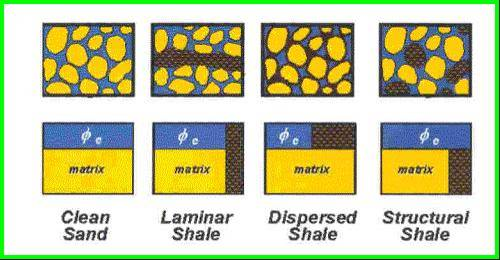Shale Volume CALCULATIONS

This page is a highly abbreviated version of Chapter 11 on this website. For more about shale, clay types, alternate methods and examples, go to the Main Index Page.

Shale is an imprecise term used to describe a rock composed of clay, silt, and bound water. The clay type and silt composition can vary considerably from one place to another. These can be determined from appropriate cross plots of PE, thorium, and potassium logs. The bound water volume varies with clay type, depth of burial, and burial history. Some shales have not lost as much water as others at similar depths and are called overpressured shales. Most shales are radioactive due to potassium and thorium, and sometimes due to uranium.Shale distribution can vary, as shown above. The shale volume calculations shown below are insensitive to the shale distribution. However, porosity and water saturation are strongly affected by laminated shale, so the methods shown in Sections 5, 6, 7, and 8 in this handbook do not apply to laminated shaly sands.

You must choose the appropriate methods for each zone, but the minimum rule works well in most cases, provided the usage rules have been honored first.CALCULATING Shale Volume

Shale volume estimation is the first calculation step in a log analysis. All other calculations depend on the shale volume being known from this step.

STEP 1: Convert density log (gm/cc or kg/m3) to porosity units if a density porosity log is not available (skip this step if density data is already in porosity units):

1: PHIDSH = (DENSSH – KD2) / (KD1 – KD2) – do this once in an obvious shale zone

2: PHID = (DENS – KD2) / (KD1 – KD2) – do this for every data level

Where:  KD1 = 1.00 for English units

KD1 = 1000 for Metric units

KD2 = 2.65 for English units Sandstone scale log

KD2 = 2650 for Metric units Sandstone scale log

KD2 = 2.71 for English units Limestone scale log

KD2 = 2710 for Metric units Limestone scale log

KD2 = 2.87 for English units Dolomite scale log

KD2 = 2870 for Metric units Dolomite scale log

NOTE: The choice for KD2 must match the neutron log units – if neutron is in Limestone units, KD2 must be 2.71 for gm/cc or 2710 for kg/m3 log scale.

STEP 2: Calculate shale volume from the three common  methods:

3: Vshg = (GR - GR0) / (GR100 - GR0)

4: Vshs = (SP - SP0) / (SP100 - SP0)

5: Vshx = (PHIN - PHID) / (PHINSH - PHIDSH)

In bitumen bearing oil sands or heavy oil, add the resistivity method:

6: Vshr = (log(RESS) - log(RMAX)) / (log(RSH) - log(RMAX))

DOES NOT work in water zones so combine this methhod with any other feasible model to handle the water zones.

In radioactive sands, replace the gamma ray method with Thorium method if gamma ray spectral data is available:

7: Vshth = (TH - TH0) / (TH100 - TH0)

NOTE: Trim Vsh values between 0.0 and 1.0. If too many values fall outside this range, check the clean and shale parameters. Do not calculate methods which fail to pass all usage rules listed below.

STEP 3: Adjust gamma ray method for young rocks, if needed:

8: Vshc = 1.7 - (3.38 - (Vshg + 0.7) ^ 2) ^ 0.5

STEP 4: Take minimum of available methods:

9: Vsh = Min (Vshg, Vshs, Vshr, Vshx, Vshc)

Calibration of log analysis shale volume is usually accomplished by comparing it to sample descriptions, core description, thin section point counts, or X-ray diffraction data.USAGE RULES:

·         Use uranium corrected gamma ray (CGR) in preference to uncorrected GR

·         Do not use GR in radioactive sandstones or carbonates. Use Thorium curve from NGT for radioactive sandstone, and uranium corrected GR (CGR) curve for radioactive carbonates.

·         Do not use SP in fresh water formations, salt mud systems, high resistivity zones, or in carbonates.

·         Do not use density neutron crossplot when bad hole, gas, or heavy minerals are present.

·         Do not use the nonlinear young rock model unless there is some evidence that it is needed.

If log analysis porosity is too low, calculated shale volume may be too high (or vice versa).

The shale in the zone may not have the same properties as nearby shales seen on the log. Therefore, some adjustments to shale properties might be necessary.

Average effective porosity calculated from logs is pessimistic in thinly laminated sand shale series, and unconventional methods should be used to determine porosity and water saturation.PARAMETERS:

GR0 = 8 to 35                           GR100 = 75 to 150

SP0 = -20 to -120                      SP100 = +20 to -20

PHIDSH = -0.06 to +0.20           PHINSH = 0.15 to 0.45

All values must be picked from logs or assumed from previous experience.

Page Views ---- Since 01 Jan 2015
Copyright 2023 by Accessible Petrophysics Ltd.
CPH Logo, "CPH", "CPH Gold Member", "CPH Platinum Member", "Crain's Rules", "Meta/Log", "Computer-Ready-Math", "Petro/Fusion Scripts" are Trademarks of the Author